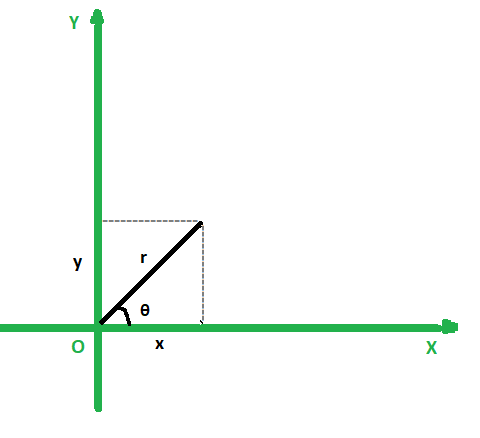# Program to convert polar co-ordinates to equivalent cartesian co-ordinates

• Last Updated : 22 Nov, 2021

Given two integers r and θ (in degree) representing polar coordinates of a point (r, θ), the task is to find the Cartesian coordinates of the given point.

Examples:

Attention reader! Don’t stop learning now. Get hold of all the important DSA concepts with the DSA Self Paced Course at a student-friendly price and become industry ready.  To complete your preparation from learning a language to DS Algo and many more,  please refer Complete Interview Preparation Course.

In case you wish to attend live classes with experts, please refer DSA Live Classes for Working Professionals and Competitive Programming Live for Students.

Input: r = 1.4142, θ = 45
Output: 1.000, 1.000

Input: r = 3, θ = 30
Output: 2.598, 1.500

Approach: Let the cartesian coordinates of the point be (x, y). The polar coordinates and the Cartesian coordinates can be related using the following equations:

x = r*cosθ and y = r*sinθFollow the steps below to solve the problem:

• Convert θ from degrees to radian as θ(in radian) = θ (in degrees) * (3.14159 / 180).
• Store the x and y coordinate in a variable X and Y respectively.
• Apply transformation formula and update the value of X = r * cosθ and Y = r * sinθ.
• Print the value of X and Y as the result.

Below is the implementation of the above approach:

## C++14

 `// C++ program for the above approach``#include ``using` `namespace` `std;` `// Function to convert degree to radian``double` `ConvertDegToRad(``double` `degree)``{``    ``double` `pi = 3.14159;``    ``return` `(degree * (pi / 180.0));``}` `// Function to convert the polar``// coordinate to cartesian``void` `ConvertToCartesian(``    ``pair<``double``, ``double``> polar)``{` `    ``// Convert degrees to radian``    ``polar.second = ConvertDegToRad(``        ``polar.second);` `    ``// Applying the formula:``    ``// x = rcos(theata), y = rsin(theta)``    ``pair<``double``, ``double``> cartesian``        ``= { polar.first * ``cos``(polar.second),``            ``polar.first * ``sin``(polar.second) };` `    ``// Print cartesian coordinates``    ``printf``(``"%0.3f, %0.3f"``,``           ``cartesian.first,``           ``cartesian.second);``}` `// Driver Code``int` `main()``{``    ``// Given polar coordinates``    ``pair<``double``,``         ``double``>``        ``polar = { 1.4142, 45 };` `    ``// Function to convert polar``    ``// coordinates to equivalent``    ``// cartesian coordinates``    ``ConvertToCartesian(polar);` `    ``return` `0;``}`

## Java

 `// Java code of above approach``import` `java.util.*;` `class` `GFG``{` `  ``// Function to convert degree to radian``  ``static` `double` `ConvertDegToRad(``double` `degree)``  ``{``    ``double` `pi = ``3.14159``;``    ``return` `(degree * (pi / ``180.0``));``  ``}` `  ``// Function to convert the polar``  ``// coordinate to cartesian``  ``static` `void` `ConvertToCartesian(``    ``double``[] polar)``  ``{` `    ``// Convert degrees to radian``    ``polar[``1``] = ConvertDegToRad(``      ``polar[``1``]);` `    ``// Applying the formula:``    ``// x = rcos(theata), y = rsin(theta)``    ``double``[] cartesian``      ``= { polar[``0``] * Math.cos(polar[``1``]),``         ``polar[``0``] * Math.sin(polar[``1``]) };` `    ``// Print cartesian coordinates``    ``System.out.print(String.format(``"%.3f"``, cartesian[``0``])+``" "``+String.format(``"%.3f"``, cartesian[``1``]));` `  ``}` `  ``// Driver code``  ``public` `static` `void` `main(String[] args)``  ``{``    ``// Given polar coordinates` `    ``double``[] polar = { ``1.4142``, ``45` `};` `    ``// Function to convert polar``    ``// coordinates to equivalent``    ``// cartesian coordinates``    ``ConvertToCartesian(polar);` `  ``}``}` `// This code is contributed by offbeat`

## Python3

 `# Python 3 program for the above approach``import` `math` `# Function to convert degree to radian``def` `ConvertDegToRad(degree):``    ``pi ``=` `3.14159``    ``return` `(degree ``*` `(pi ``/` `180.0``))` `# Function to convert the polar``# coordinate to cartesian``def` `ConvertToCartesian(polar):` `    ``# Convert degrees to radian``    ``polar[``1``] ``=` `ConvertDegToRad(polar[``1``])` `    ``# Applying the formula:``    ``# x = rcos(theata), y = rsin(theta)``    ``cartesian ``=` `[polar[``0``] ``*` `math.cos(polar[``1``]),``                 ``polar[``0``] ``*` `math.sin(polar[``1``])]` `    ``# Print cartesian coordinates``    ``print``(``'%.3f'` `%` `cartesian[``0``],``          ``'%.3f'` `%` `cartesian[``1``])` `# Driver Code``if` `__name__ ``=``=` `"__main__"``:` `    ``# Given polar coordinates``    ``polar ``=` `[``1.4142``, ``45``]` `    ``# Function to convert polar``    ``# coordinates to equivalent``    ``# cartesian coordinates``    ``ConvertToCartesian(polar)` `    ``# This code is contributed by chitranayal.`

## Javascript

 ``
Output:
`1.000, 1.000`

Time Complexity: O(1)
Auxiliary Space: O(1)

My Personal Notes arrow_drop_up